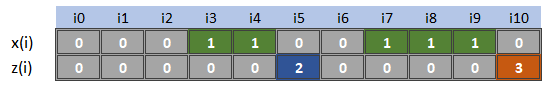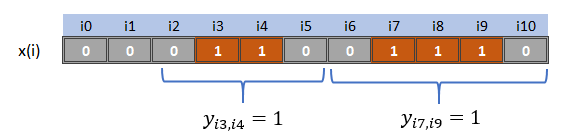## Friday, April 13, 2018

### A difficult MIP construct: counting consecutive 1's

In  following modeling question is posed:

Given binary variables $$x_i \in \{0,1\}$$, I want to form an integer variable $$z_i$$ that has the length of the preceding string of ones (this is sometimes called the run-length). I.e.:Given x(i) find z(i)
This is not so easy to do. Here is my attempt. The idea behind my approach, is to use another intermediate integer variable $$a_i$$ (accumulator) defined by $a_i = \begin{cases} a_{i-1}+1 & \text{if x_i=1 }\\ 0 & \text{otherwise}\end{cases}$Additional variable a(i)

#### Step 1: form $$a_i$$

The calculation of variable $$a_i$$ can be stated as a quadratic constraint: $a_i = x_i(a_{i-1}+1)$

In terms of indicator constraints (implications as available in many MIP solvers) this would look as follows: \begin{align} &x_i=0 \Rightarrow a_i = 0\\ &x_i = 1 \Rightarrow a_i = a_{i-1}+1\end{align}

If the MIP solver does not support indicator constraints, we can write this as a bunch of linear inequalities: \begin{align} & (a_{i-1}+1) - (1-x_i)M \le a_i \le (a_{i-1}+1) + (1-x_i)M\\ & 0 \le a_i \le x_i M \end{align} Here $$M$$ is the length of the largest possible string of consecutive 1's, e.g.$$M=\mathit{card}(i)$$.

We can also linearize the quadratic constraint, but that leads to extra variables.

#### Step 2: form $$z_i$$

To go from $$a_i$$ to $$z_i$$, we can define: $z_i = \begin{cases} a_{i-1} & \text{if x_i=0 and x_{i-1}=1 }\\ 0 & \text{otherwise}\end{cases}$ In implication form this is:\begin{align} & x_i=0 \text{ and } x_{i-1}=1 \Rightarrow z_i =a_{i-1}\\ & x_i=1 \text{ or } x_{i-1}=0 \Rightarrow z_i=0 \end{align} This is not immediately ready for use with indicator constraints (these constraints want a single binary variable at the left of $$\Rightarrow$$). We can introduce a new binary variable $$y_i \in \{0,1\}$$  and linearize $$y_i = x_{i-1} (1-x_i)$$ using: $y_i = x_{i-1} (1-x_i) \Leftrightarrow \begin{cases} y_i \le x_{i-1}\\ y_i \le 1-x_i\\ y_i \ge x_{i-1} - x_i\\ y_i \in \{0,1\}\end{cases}$ (We can relax $$y_i$$ to $$y_i \in [0,1]$$). Now we are able to implement the indicator constraints:\begin{align}&y_i \ge x_{i-1}-x_i\\ &y_i \le x_{i-1}\\ & y_i \le 1-x_i\\ & y_i=1 \Rightarrow z_i =a_{i-1}\\ & y_i=0 \Rightarrow z_i=0 \\& y_i \in \{0,1\} \end{align}

Written as linear inequalities, we have: \begin{align} & a_{i-1} - x_i M - (1-x_{i-1})M \le z_i \le a_{i-1} + x_i M + (1-x_{i-1})M\\ & 0 \le z_i \le 1-x_{i-1} M \\& z_i\le (1-x_i)M \end{align}

An MINLP formulation is simpler: $z_i = a_{i-1}(1-x_i) x_{i-1}$

#### Putting things together

To summarize: we can come up with (at least) three different formulations:

big-M inequalitiesIndicator constraintsMINLP formulation
\begin{align}&a_i \le (a_{i-1}+1) + (1-x_i)M\\ & a_i \ge (a_{i-1}+1) - (1-x_i)M\\& a_i \le x_i M\\& z_i \le a_{i-1} + (1-x_{i-1}+x_i)M\\& z_i \ge a_{i-1} - (1-x_{i-1}+x_i)M \\ &z_i \le x_{i-1} M\\ & z_i\le (1-x_i)M\\& a_{i} \in \{0,1,2,\dots\}\\ & z_{i} \in \{0,1,2,\dots\} \end{align} \begin{align} & x_i=0 \Rightarrow a_i = 0\\ &x_i = 1 \Rightarrow a_i = a_{i-1}+1\\ & y_i \le 1-x_i\\& y_i \le x_{i-1}\\ &y_i \ge x_{i-1}-x_i \\ & y_i = 0 \Rightarrow z_i = 0\\& y_i=1 \Rightarrow z_i = a_{i-1}\\ &a_{i} \in \{0,1,2,\dots\}\\ &y_{i} \in \{0,1\} \\ & z_{i} \in \{0,1,2,\dots\} \end{align} \begin{align} &a_i = x_i(a_{i-1}+1)\\ &z_i = a_{i-1}(1-x_i) x_{i-1}\\& a_{i} \in \{0,1,2,\dots\}\\ & z_{i} \in \{0,1,2,\dots\} \end{align}

This is complex enough, we really have to try this out. The results of the big-$$M$$ inequality approach look like:

----     71 VARIABLE x.L

i3 1.000,    i4 1.000,    i7 1.000,    i8 1.000,    i9 1.000

----     71 VARIABLE a.L

i3 1.000,    i4 2.000,    i7 1.000,    i8 2.000,    i9 3.000

----     71 VARIABLE z.L

i5  2.000,    i10 3.000


The MINLP formulation is the most elegant: just two equations.

#### Can we do this without intermediate variables $$a_i$$?

Yes, at the expense of more nonzero elements in the LP matrix. We can define: $z_i = \begin{cases} \displaystyle \sum_{k\lt i} x_k - \displaystyle \sum_{k\lt i} z_k & \text{if x_i=0 and x_{i-1}=1 }\\ 0 & \text{otherwise}\end{cases}$z(i10) = green cells − blue cells

In big-$$M$$ inequality form we can write: \begin{align} & \sum_{k\lt i} (x_k - z_k) - (1-x_{i-1}+x_i)M \le z_i \le \sum_{k\lt i} (x_k -z_k) + (1-x_{i-1}+x_i)M\\ & 0 \le z_i \le 1-x_{i-1} M \\& z_i\le (1-x_i)M \end{align} I prefer the versions with extra variables $$a_i$$.

#### A stronger formulation

In the comments of  a much stronger formulation is proposed: no big-$$M$$'s needed. A disadvantage is that we need many variables ($$n^2/2$$) and even more constraints. The idea is to find occurrences of a run starting at position $$i$$ and ending at position $$j$$.y(i,j)=1 represents a run from i to j

Such a run can be found by: $y_{i,j}=(1-x_{i-1})\left(\prod_{k=i}^j x_k\right) (1-x_{j+1})$ This is a product of binary variables. The basic linearization is: $y=x_1 x_2 \Leftrightarrow \begin{cases} y \le x_1\\ y \le x_2\\ y \ge x_1+x_2-1\\ y\in \{0,1\}\end{cases}$ This formulation allows us to relax $$y\in \{0,1\}$$ to $$y\in [0,1]$$  Generalizing this, leads to:\begin{align}&y_{i,j} \le 1-x_{i-1}\\&y_{i,j} \le x_{k}&& k=i,\dots,j\\ &y_{i,j} \le 1-x_{j+1}\\&y_{i,j}\ge \sum_{k=i}^j x_k - x_{i-1}-x_{j+1} - (j-i)\\ &y_{i,j}\in \{0,1\} && j\ge i\\ \end{align} Again, if we want, we can relax $$y_{i,j}$$ to be continuous between zero and one. With these $$y_{i,j}$$ we can calculate $$z_j$$ as:$z_j = \sum_{i\le j} (j-i+1)y_{i,j}$

When we try this out we see:

----    134 VARIABLE x.L

i3 1.000,    i4 1.000,    i7 1.000,    i8 1.000,    i9 1.000

----    134 VARIABLE y.L

i4          i9

i3        1.000
i7                    1.000

----    134 VARIABLE z.L

i4 2.000,    i9 3.000


For our small example with $$n=\mathit{card}(i)=11$$ we end up with a total of 88 variables (66 are $$y_{i,j}$$'s) and 495 constraints. If we would have $$n=\mathit{card}(i)=100$$ these numbers increase to 5,250 variables and 186,950 equations. The number of equations is roughly $$n^3/6$$.

With some invented models, this formulation did not seem competitive with the earlier MIP models. There may well be models where this formulation work better. Modern MIP solvers are very good in generating cuts. This means that "smaller" (fewer variables and equations) but weaker formulations are not so bad anymore: the solver will strengthen the model as it goes.

#### Maximum run-length

I doubt the user really needs this. Usually if we know more about the rest of the problem, we can simplify things considerably. For instance, suppose the model really only needs to establish an upper bound on the run-length. If we only need $$z_i \le R$$, we can drop a boatload of constraints. One formulation can be: \begin{align} & x_i =1 \Rightarrow z_i = z_{i-1} + 1\\ & z_i \le R\end{align} A formulation only involving the original $$x_i$$ variables can be: $\sum_{k=i}^{i+R}x_k \le R \>\> \forall i$

This is a good example where we need to look at the whole model to give the best possible advice on how to model things. Just looking at a fragment may lead to formulations that are too general and thus way too complicated.

#### References

1. Linear/Integer Programming: Counting Consecutive Ones, https://math.stackexchange.com/questions/2732897/linear-integer-programming-count-consecutive-ones